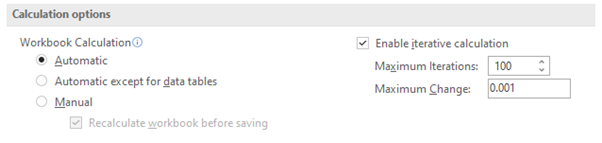## What is Sensitizing DCF Analysis for Key Variables?

A discounted cash flow (DCF) analysis is highly sensitive to key variables such as the long-term growth rate (in the growing perpetuity version of the terminal value) and the weighted average cost of capital (WACC) . As a result, it is important to sensitize the output for these key variables to provide a valuation range.

Sensitivity tables allow for a range of values to be quickly calculated based and can be built manually or using Excel’s data table functionality.

## Key Learning Points

• DCF analysis is highly sensitive to some of the key variables such as the long-term growth rate (in the growing perpetuity version of the terminal value) and the WACC
• It is critical that the output of DCF analysis is sensitized for key variables to provide a valuation range
• Sensitizing key variables help to understand the sensitivity of the DCF model to key assumptions
• Sensitivity tables can be built manually or using Excel’s data table functionality. Data tables allow dynamic sensitivities to multiple assumptions

## The Need for Sensitizing

A DCF analysis calculates the enterprise value of a business as the present value of its forecasted free cash flows. A strength of DCF analysis is the requirement to think about and forecast key business drivers. However, DCF valuation is very dependent on key assumptions with even small changes producing large value variations. Sensitizing key variables help in understanding the sensitivity of the DCF model to key assumptions.

## Example: Sensitizing Key Variables for DCF

Below we have been provided information about the inputs used in a DCF analysis and the resulting enterprise value. In this DCF analysis, the WACC and long-term growth rate assumptions are the variables being sensitized. Attached to this blog we have included the full DCF analysis.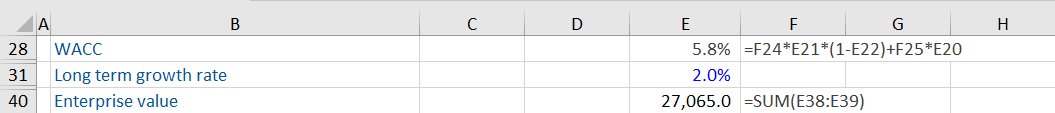The WACC assumption has been calculated and the long-term growth rate has been provided as a fixed assumption.

Sensitivity tables can be built manually or using Excel’s data table functionality. The data table functionality in Excel is memory intensive so often analysts will turn the calculation setting to ‘Automatic except tables’. If you change the calculation setting you can calculate the data tables by pressing the F9 key.

We use the data table feature of Microsoft Excel to perform this sensitivity analysis. In a two-way data table, the information is organized as follows: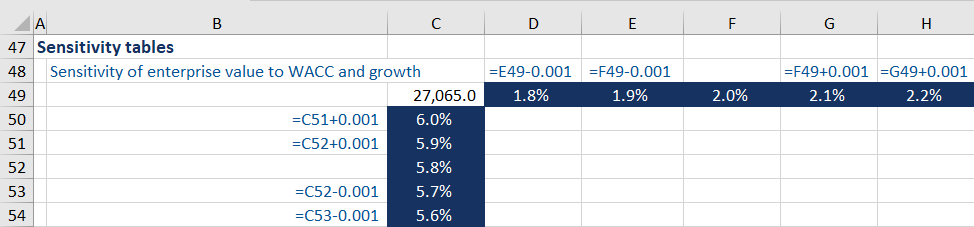The link reference to the output cell being sensitized (in this case the enterprise value) is entered in cell C49. In this case, the Enterprise Value is in cell E40. The variable growth has been entered in rows (D49:H49) while the variable WACC has been inserted in columns (C50:C54) of the table. The values in cells F49 and C52 are hardcoded and are the mid points. The remaining incremental assumptions are added using a formula. While there is a workaround so you can use hard numbers in the assumptions of a data table it is not intuitive and not well used so we are not covering it here.

The area inside the table (D50:H54) is the answer area. Excel will substitute each variable combination into the formula being sensitized and return each possible answer in this area.

To create the data table, select the area which includes both the row and column variables (C50:H54). To activate the data table dialogue box, we use the keyboard shortcut Alt A W T (from the Data Ribbon and What If Analysis area).

The row input cell is the long-term growth rate assumption from cell E31 (the ‘row’ along the top of the data table), and the column input cell is WACC from cell E28 (the ‘column’ along the left of the data table). An easy way to remember which reference to put into which cell in the dialogue box is the top box relates to the assumption being sensitized across the top of the data table.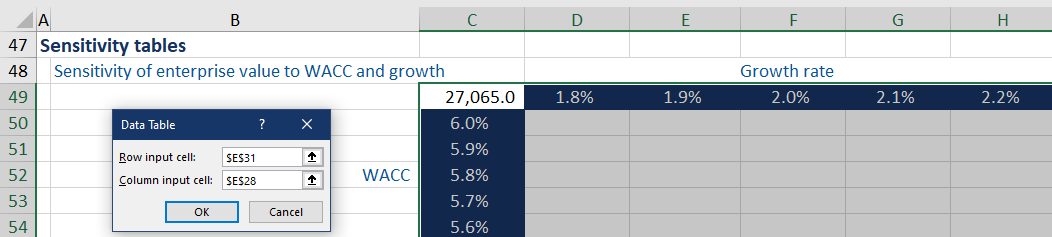Upon pressing OK, we get the following as results of the calculation: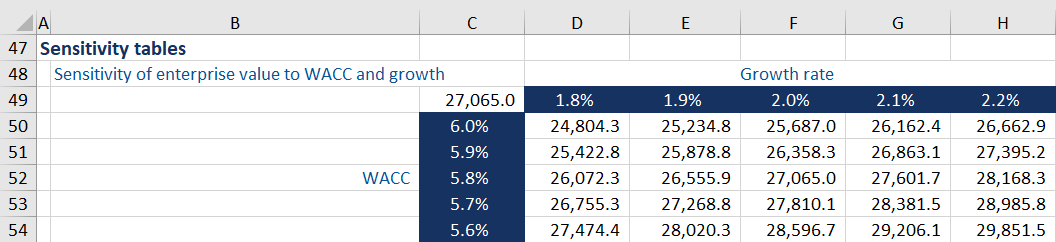The bottom right corner of the table uses the highest long-term growth rate of 2.2%. When combined with the lowest WACC of 5.6%, it gives the highest enterprise value of 29,851.5.

The midpoint of using 2.0% as the long-term growth rate and 5.8% as the WACC gives the same enterprise value 27,065.0 as the model answer.

The top left of the table that combines the lowest growth rate 1.8% and the highest WACC 6.0% gives the lowest enterprise value of 24,804.3.

While this is an example, a variety of outputs can be sensitized for different variables. The findings can lead to valuable insights for analysts and investors. It’s preferable to build the data table so the lowest value is in the top left-hand corner, and the highest value is in the bottom right-hand corner.

As seen above, DCF valuations are highly sensitive to changes in input variables. There are a few very important issues here:

• Avoid wide ranges in the underlying assumptions for the long-term growth rate and WACC. We would recommend not sensitizing these numbers by more than 0.5%
• Changing the growth rate in the terminal value assumption in most DCF models does not make a corresponding adjustment to the amount of capital invested in net PP&E and working capital. When we are changing the growth rate we are sensitizing how much growth the business can support given an investment in net PP&E and working capital
• If you want to use a wider range of growth sensitivities we suggest you use the value driver formula for the terminal value

The images above are snapshots of a DCF model. Download the workout from the link for a full breakdown of the DCF model used.

In case you are not getting the desired results, make sure to check the “Formula” settings in Excel. These can be accessed using the shortcut ALT F T. The “Workbook Calculation” must be set as “Automatic” so the data table can automatically update.